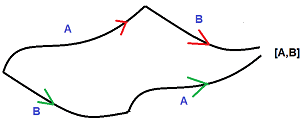# Applications of measurement of commutativity

• I
In summary, the commutator is an operation on two linear operators that measures the gap between flowing along the two operators in different orders. It is often used as a heuristic for measuring the commutativity of matrices, but it needs to be defined on a curved space to be useful. This has a fundamental relationship to trajectories and derivatives on manifolds, as explained in the link provided.

Dang this place has a topology and analysis section too, nice.

This is probably a graduate level topic, but I am by no means an expert on these subjects, just things I learn from wikipedia and other people. The commutator is an operation on two linear operators (most often matrices) of the form ##[A,B] = AB - BA.## This is often touted as a measurement of how "badly" two matrices fail to commute, but I don't think it quite is.

I'd like to research what happens when we induce a matrix norm to the commutator, when the matrix norm of the commutator ##|| [A,B] ||^2## actually returns a specific number, I think that's more of a "measurement" of the commutativity of two matrices.

However, why bother? Are there any theoretical or scientific applications for measuring the size of matrix commutators? Possibly in quantum physics, though I don't know that subject in depth, I hope there are more applications than that.

Last edited:
You have to consider ##A## and ##B## as vector fields. ##[A,B]## measures the gap you get if you flow along ##A## and then ##B##, or the other way around. It doesn't measure how badly matrices commute, it measures how commutative flows along vector fields are.

•topsquark
Okay, that's interesting. What about measuring the commutativity of matrices instead of vectors though? I haven't seen the commutator in the case that ##A## and ##B## are vector fields, but rather matrices.•